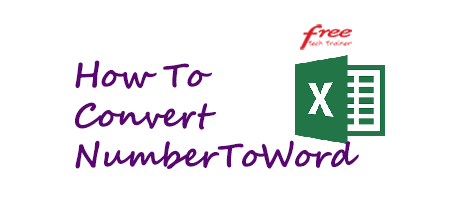# How To Convert Number To Word in ExcelIn our professional life, sometimes we need to convert number value into word in excel. You can do this by creating a macro. Today I’ll show you how to do that in 5 easy steps:

Step-1:
Press alt+F8 to open Visual Basic Editor. If your macro is disable you have to enable your macro first.

Step-2:
Click the Insert Tab and then click Module

Step-3:
Copy the following code into the module window

```Option Explicit

Function NumberToWord(ByVal aNumber)
Dim Takas, Paisa, Temp
Dim DecimalPlace, Count
ReDim Place(9) As String
Place(2) = " Thousand "
Place(3) = " Million "
Place(4) = " Billion "
Place(5) = " Trillion "
aNumber = Trim(Str(aNumber))
DecimalPlace = InStr(aNumber, ".")
If DecimalPlace > 0 Then
Paisa = GetTens(Left(Mid(aNumber, DecimalPlace + 1) & "00", 2))
aNumber = Trim(Left(aNumber, DecimalPlace - 1))
End If
Count = 1
Do While aNumber <> ""
Temp = GetHundreds(Right(aNumber, 3))
If Temp <> "" Then Takas = Temp & Place(Count) & Takas
If Len(aNumber) > 3 Then
aNumber = Left(aNumber, Len(aNumber) - 3)
Else
aNumber = ""
End If
Count = Count + 1
Loop
Select Case Takas
Case ""
Takas = "No Takas"
Case "One"
Takas = "One Taka"
Case Else
Takas = Takas & " Takas"
End Select
Select Case Paisa
Case ""
Paisa = " and No Paisa"
Case "One"
Paisa = " and One Cent"
Case Else
Paisa = " and " & Paisa & " Paisa"
End Select
NumberToWord = Takas & Paisa
End Function

Function GetHundreds(ByVal aNumber)
Dim Result As String
If Val(aNumber) = 0 Then Exit Function
aNumber = Right("000" & aNumber, 3)
If Mid(aNumber, 1, 1) <> "0" Then
Result = GetDigit(Mid(aNumber, 1, 1)) & " Hundred "
End If
If Mid(aNumber, 2, 1) <> "0" Then
Result = Result & GetTens(Mid(aNumber, 2))
Else
Result = Result & GetDigit(Mid(aNumber, 3))
End If
GetHundreds = Result
End Function

Function GetTens(TensText)
Dim Result As String
Result = ""
If Val(Left(TensText, 1)) = 1 Then
Select Case Val(TensText)
Case 10: Result = "Ten"
Case 11: Result = "Eleven"
Case 12: Result = "Twelve"
Case 13: Result = "Thirteen"
Case 14: Result = "Fourteen"
Case 15: Result = "Fifteen"
Case 16: Result = "Sixteen"
Case 17: Result = "Seventeen"
Case 18: Result = "Eighteen"
Case 19: Result = "Nineteen"
Case Else
End Select
Else
Select Case Val(Left(TensText, 1))
Case 2: Result = "Twenty "
Case 3: Result = "Thirty "
Case 4: Result = "Forty "
Case 5: Result = "Fifty "
Case 6: Result = "Sixty "
Case 7: Result = "Seventy "
Case 8: Result = "Eighty "
Case 9: Result = "Ninety "
Case Else
End Select
Result = Result & GetDigit _
(Right(TensText, 1)) '
End If
GetTens = Result
End Function

Function GetDigit(Digit)
Select Case Val(Digit)
Case 1: GetDigit = "One"
Case 2: GetDigit = "Two"
Case 3: GetDigit = "Three"
Case 4: GetDigit = "Four"
Case 5: GetDigit = "Five"
Case 6: GetDigit = "Six"
Case 7: GetDigit = "Seven"
Case 8: GetDigit = "Eight"
Case 9: GetDigit = "Nine"
Case Else: GetDigit = ""
End Select
End Function
```

Step-4:
Click Save button and press Alt+Q button to return to the excel. Your defined function is now ready to use.You can use this function as other built-in function. i.e. There is value 9,216,857.34 in c20 column. You want to convert it into word at c21 column. So, write =NumberToWord(c20) and press Enter. You will see “Nine Million Two Hundred Sixteen Thousand Eight Hundred Fifty Seven Takas and Thirty Four Paisa” at c21.

Step-5:
Last step is to save your file with macro. Click File then Click Save As and Then select Excel Macro-Enabled Workbook (.xlsm) and click Save. Your work is done. Now you can reuse the file.

If you like my post like my facebook page http://www.facebook.com/freetechtrainer/. Be with us and hope you can learn many things.